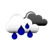### Factorial Calculator

 Example: Factorial for 3 = 3! = 3 x 2 x 1 = 6 Factorial for: Answer:

The Factorial Calculator tool enables you to calculate the factorial of a chosen number.

In maths, factorial means to multiply all whole numbers from the chosen number down to 1.

#### The Factorial Formula

You can use the factorial formula to find the factorial of a number:

n! = n × (n-1) × (n-2) ×...× 1

Example:

5! = 5 × 4 × 3 × 2 × 1 = 120

#### References

More references for Factorial

#### Local Weather Status

Ashburn, United States
9th December, 2019 Monday
Light Intensity Drizzle6.67 - 9.44
Humidity: 87 %
Wind: 4.6 km/h